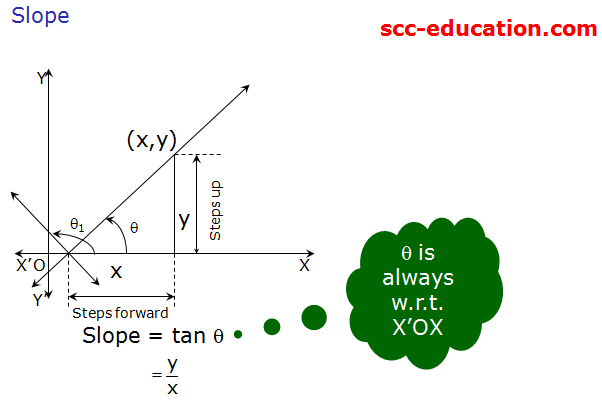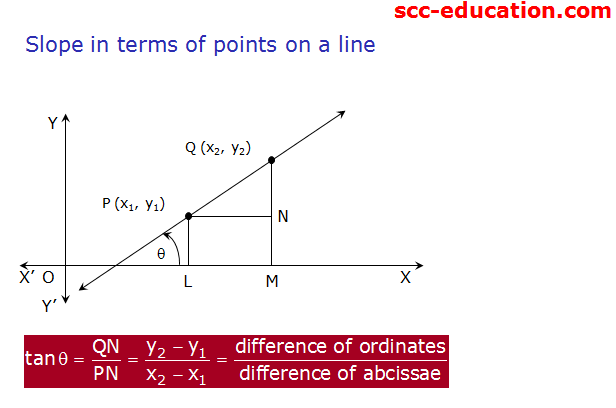# SCC Education

## Cartesian Coordinate Geometry and Straight Lines

Cartesian Coordinate Geometry
and Straight Lines
Session Objectives
1.Definition of straight line – locus
2.Slope of a line
3.Angle between two lines
4.Intercepts of a line on the axes
5.Slope, intercept form
6.Point, slope form
7.Two-point form
8.Intercepts form
9.Normal form
10.Parametric or distance form
Locus definition of a straight line
A straight line is the locus of a point whose coordinates satisfy a linear equation